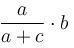Courses

# Centre Of Mass MCQ Level - 1

## 10 Questions MCQ Test Basic Physics for IIT JAM | Centre Of Mass MCQ Level - 1

Description
This mock test of Centre Of Mass MCQ Level - 1 for Physics helps you for every Physics entrance exam. This contains 10 Multiple Choice Questions for Physics Centre Of Mass MCQ Level - 1 (mcq) to study with solutions a complete question bank. The solved questions answers in this Centre Of Mass MCQ Level - 1 quiz give you a good mix of easy questions and tough questions. Physics students definitely take this Centre Of Mass MCQ Level - 1 exercise for a better result in the exam. You can find other Centre Of Mass MCQ Level - 1 extra questions, long questions & short questions for Physics on EduRev as well by searching above.
QUESTION: 1

### A particle of mass m moving with velocity u1 collides elastically with another particle of same mass moving with velocity u2 in the same direction. After collision their speeds are v1 and v2 respectively then (A) u1 + v1 = v2 + u2 (B) u1 – v1 = v2 + u2

Solution:

Equation A is correct but not B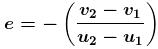(For elastic collision e = 1)
⇒ 1(u2 – u1) = –(v2 – v1)
⇒ u2 + v2 = u1 + v1
The correct answer is: Equation A is correct but not B

QUESTION: 2

### The centre of the mass of the shaded portion of the disc is : (The mass is uniformly distributed in the shaded portion) :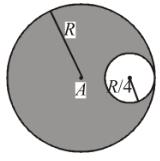Solution: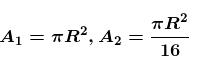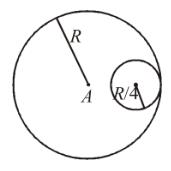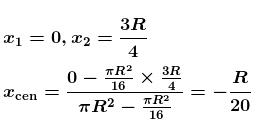The correct answer is: R/20 to the left of A

QUESTION: 3

### A man of mass M hanging with a light rope which is connected with a balloon of mass m. The system is at rest in air. When man rises a distance h with respect to balloon. Find the distance raised by man :

Solution:

Initially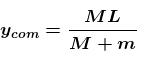since no external force is acting

∴ COM should be at rest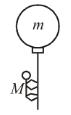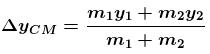Let balloon descend by a distance x.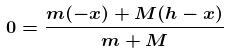Mh = (m + M)x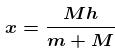(Distance descend by balloon)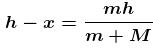(Distance raised by man)

The correct answer is: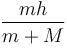QUESTION: 4

The block of mass M moving on the frictionless horizontal surface collides with the spring of spring constant k and compresses it by length L. The maximum momentum of the block after collision is :

Solution:

According to conservation of energy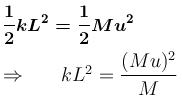MkL2 = p2     (p = mu)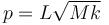The correct answer is: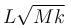QUESTION: 5

A particle of mass 4m which is at rest explodes into three fragments. Two of the fragments each of mass  m  are found to move with a speed v each in mutually perpendicular directions. The minimum energy released in the process of explosion is :

Solution: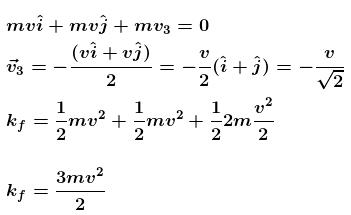The correct answer is: (3/2) mv2

QUESTION: 6

Find the position of centre of mass of the uniform planar sheet shown in figure with respect to the origin (O) :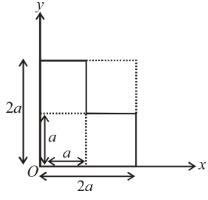Solution: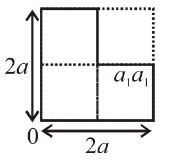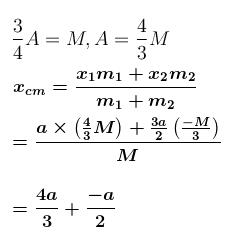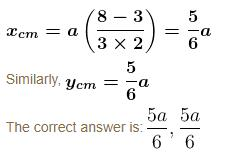QUESTION: 7

A ball of mass m strikes the fixed inclined plane after falling through a height h. If it rebounds elastically, the impulse on the ball is :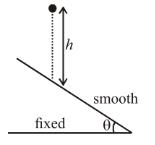Solution:

Here e = 1
If ball rebounded elastically
vLOI = uLOI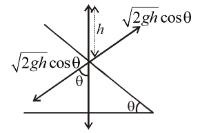∴ Along line of impact momentum conservation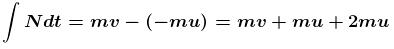Along line of impact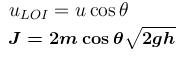The correct answer is: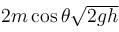QUESTION: 8

The spacecraft of mass M moves with velocity V in free space at first, then it explodes breaking into two pieces. If after explosion a piece of mass m comes to rest, the other piece of space craft will have :

Solution: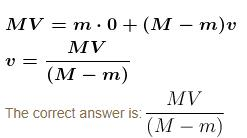QUESTION: 9

A ball impinges directly on a similar ball at rest. The first ball is brought to rest by the impact. If half the kinetic energy is lost by impact. What is the value of the coefficient of restitution?

Solution:

QUESTION: 10

A bullet of mass a  and velocity b is fired into a large block of mass c. The final velocity of the system is :

Solution:

From momentum conservation Pi = Pf
ab = (a + c)v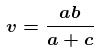The correct answer is: